Testing Berry phase method in VASP and QE for 2D system

The VASP and QE both have berry phase module that calculate the electronic polarization “automagically”. However, their results always seem to be puzzling, especially for low dimensional systems. In order to get a clear picture of how to these routine perform in 2D systems and to show how to use them correctly, a 2D NbN system and a 3D BaTiO3 structure were employed here as an example. To confirm their results, a charge-center method was also employed.

Before diving into things, let review some basic concepts that are of paramount importance to later calculations.

Background

According to the modern theory of polarization, the electrical polarization depends on the difference of dipole moment between centro-symmetric structure and non-centro-symmetric stucture. The reason behind is that the polairzation cannot be uniquely determined due to periodical boundary conditions.(That is, translation of unitcell vector can yield unphysical movements in the charge centers.)

To illustrate this, first, consider a imaginary one dimensional atomic chain that have two differenc atoms: a anion and a cation.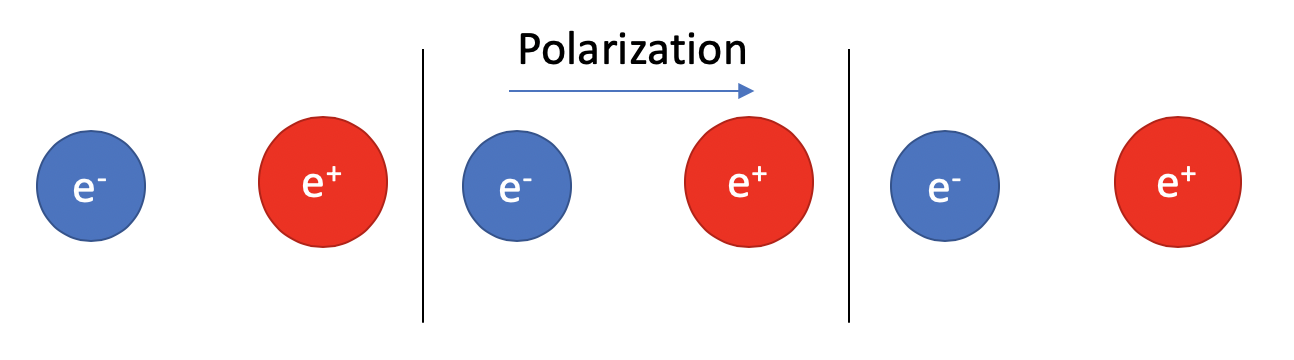We can easily determine the dipole moment pointing from the anion to the cation.

However, the physical world does not work in such simple way. If we take a step further, differentiate the electrons and ions, the problem becomes much harder to solve: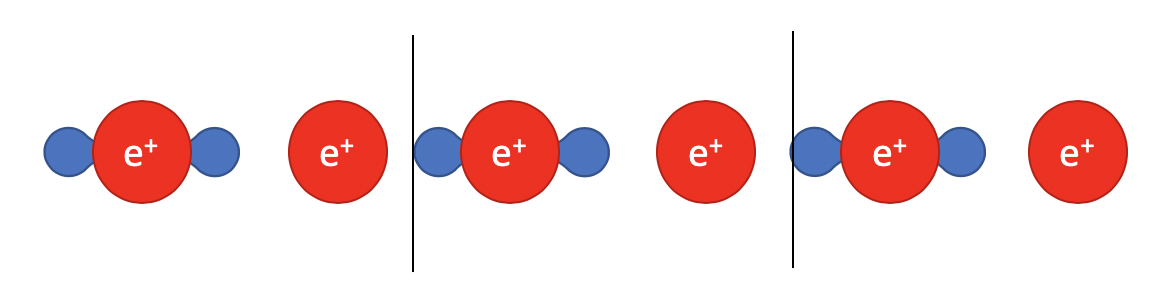Here, the electrons are represented as charge density and are spread out between two ions. To get the charge center for electrons, we have to integrate the charge density in the unitcell:

$\langle r_{electron} \rangle = \int_{unitcell}dr*\rho(r)$

And the positive charge center can be found:

$\langle R_{ion} \rangle = \Sigma_{i=A,B} R*Q_{i} /Q_{total}$

These expressions are easy to solve, but the charge-center varies with the choice of unicell. For example, if we translate the unitcell by a fraction to the right: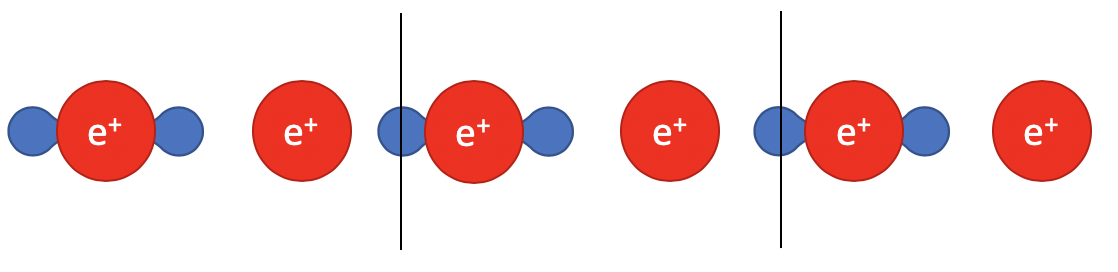Taking a closer look at the charge distribution, some of which “jumped” from the left end to the right end due to periodical conditions. And even though the structure is unchanged, this translation motion changes the charge center of electrons while left the ion center unaffected.

To eliminate this ambiguity, Vanderbilt and others found out that it is not the dipole moment for non-centrosymmetric phase that matters, the actual polarization depends on the change of polarization from the non-centrosymmetric phase to the centro-symmetric phase.

Side Note: how to define a centrosymmetric phase?

Answer: Calculate optimum transition route and extract the local maxima as centrosymmetric phase.

VASP 2D System

2D-NbN, as an out of plane ferroelectric system suggested by Anuja et al, have exotic switchable out-of-plane (OOP) polarization which can happen without switching of ionic positions. I choose this to show how Berry phase method can, sometimes, be cumbersome and have boundaries in certain systems.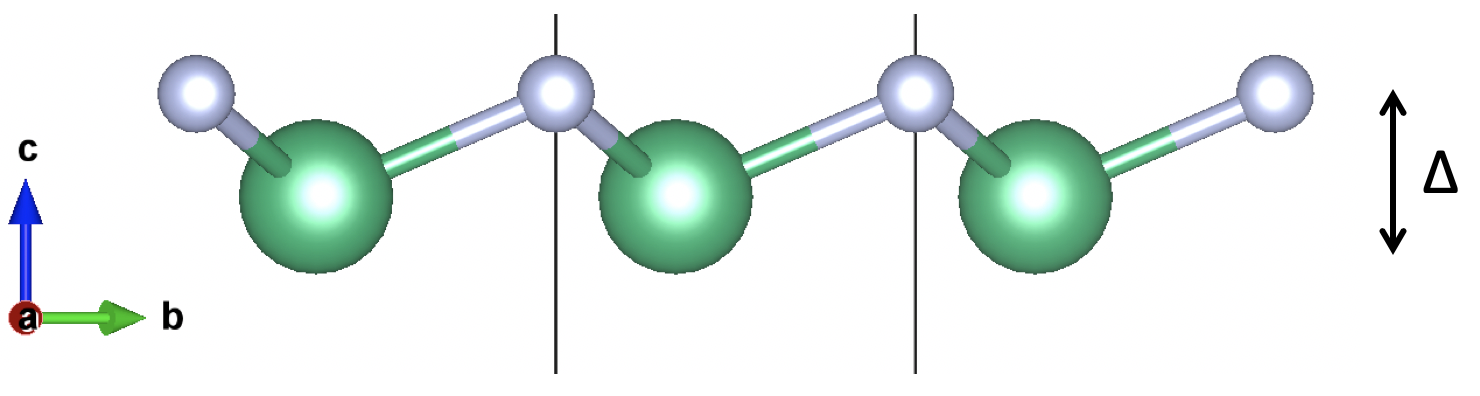The system consist of one Nb and one N atom that forms a Boron Nitride like structure. The polarization can be switched by switching the $\Delta$ in the z axis:

$\Delta=Z_{Nb}-Z_{N}$

Due to the fact that this structure possess OOP polarization in which the periodical conditions are broken, the charge center can be uniquely defined since the charge density reverts to zero at the vacuum region.

The vacuum region is chosen as around 30 Angstrom with an atrifical dipole layer so that imaging counterpart has no effects.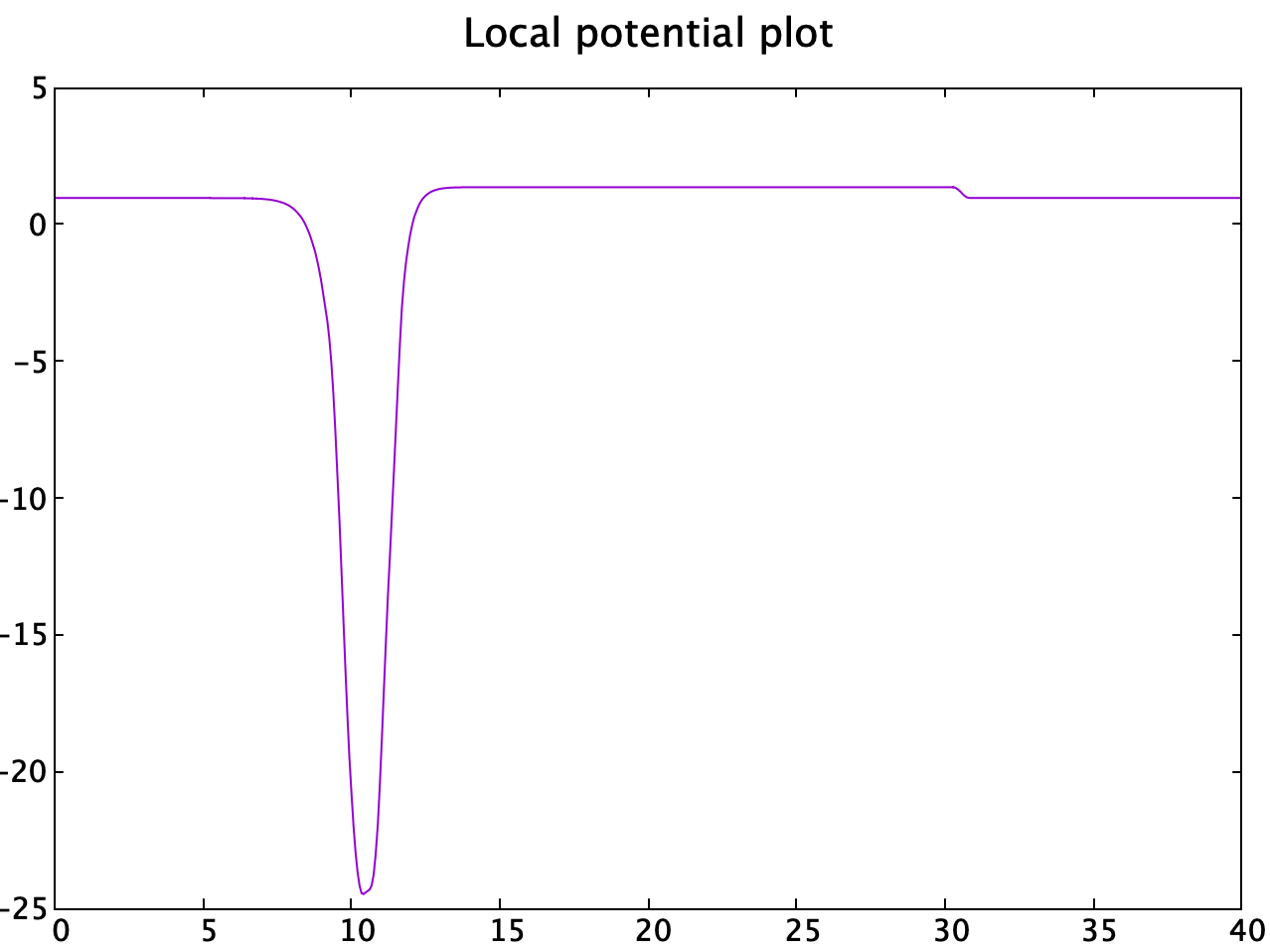Charge Center method

The charge center method is performed using this tool (link). The final polarization obtained for intrinsic NbN monolayer is:

$Polarization=3.28 pC/m$

And the transition curve is obtained almost identical to the published result: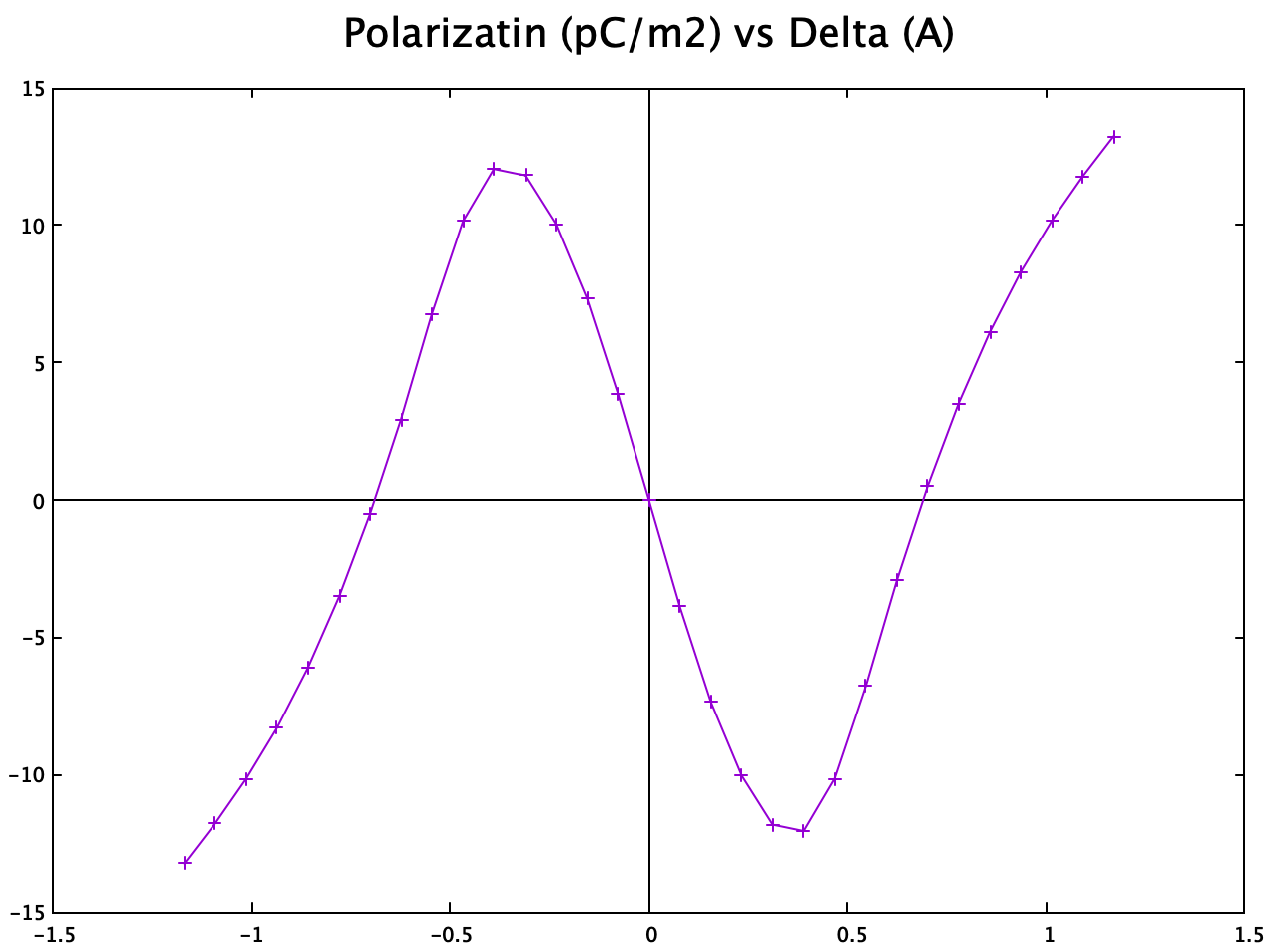This confirms the “correctness” of my charge center method even though in paper they used the berryphase method.

berryphase

To avoid error as mentioned inlink, I performed both automatic and manual berryphase calculation using the tag:

LDIPOL = .TRUE.
IDIPOL = 3
DIPOL = 0.5 0.5 0.2681174992499997
LCALCPOL = .T.

And

LDIPOL = .TRUE.
IDIPOL = 3
DIPOL = 0.5 0.5 0.26378659
LBERRY = .TRUE.
IGPAR  = 3
NPPSTR = 1

The final results does not make sense as:

Ionic dipole moment: p[ion]=(   -10.24777     5.91655     0.00000 ) electrons Angst

Total electronic dipole moment: p[elc]=(    -3.63403     2.09811    29.94773 ) electrons Angst

And

e<r>_ev=(     0.00209     0.00028    -0.01672 ) e*Angst
e<r>_bp=(     0.00000     0.00000    29.96212 ) e*Angst

Total electronic dipole moment: p[elc]=(     0.00209     0.00028    29.94540 ) e*Angst

ionic dipole moment: p[ion]=(    -6.30632    12.74335     0.00000 ) e*Angst

No matter how I tried: a different K-points, a different DIPOL value … the p[elc] does not change and stays at around 30 e*Angst. One thing that I notice: changing DIPOL tag greatly affects the convergence (mostly due to add in dipole corrections) and p[ion] value. But it does absolutely nothing to p[elc] which is puzzling.

Think of it this way, if we can define the origin of the cell using DIPOL and the electronic polarization does not change with this tag, how can we confidently say adding the electronic part to the ionic part is the right way to obtain the total polarization?

As of now, I don’t know how to use VASP’s berry phase routine to calculate electronic polarization on the non-periodical direction (OOP).

VASP 3D system

Berryphase

Now lets consider a prototypical $BaTiO_{3}$ unitcell:

By varying the atomic displacement from the centrosymmetric phase (CENT) to ferroelectric one (FE). VASP produce a series of ionic and electronic contribution to the total dipole moment.

Image Ion (elect*A) Electron (elect*A)
0 (CENT) +00.00000 +0.00000
1 -12.01278 -0.29138
2 -11.92507 -0.58047
3 -11.83735 -0.86532
4 -11.74963 -1.14461
5 -11.66192 -1.41757
6 (FE) -11.5742 -1.68399

It is clear that the centrosymmetric one has a wrong ionic contribution to the total polarziation.

At first, I thought this is due to the imposed symmtry constraint. However, after switch off symmetry entirely (ISYM=-1), VASP still produce this abnormal value of 0.0 elect*A for centrosymmetric phase.

2019-12-25 update

This abnormal value of 0.0 corresponds to the jump of oxygen atom located at the boundary of the unit cell. Specifically:

(Fractioanl coordinates)

O @ CENT : 0.5  0.5  0.0

O @ FE   : 0.5  0.5  0.975

since oxygen pseudopotential contains 6 valence electrons, we can easily calculate its ionic dipole moment at centrosymmetric phase should be 24.2 elect * Ang. Since VASP output unit in elect * Ang, we have to account for electrons negative sign, so the ionic polarization @ CENT = -24.2 elect * A

This sill doesn’t solve the mystery.

2021-05-28 update

This is actually a but in VASP, see this post.

After figure out which value is clearly wrong, we can now proceed to calculate total polarization

Total ionic contribution = 0.5258 elect*A
Total electronic contribution = -1.68399 elect*A
Total dipole moment = -1.15819 elect*A
Volume = 64.35864801 A^3

Total polarization = 0.288293871 C/m^2

Note we have to account for the negative sign of electron here. To do so, I added the negative sign to the final result.

Exactly the same withlink and experimental value of 0.26 C/m^2.

By far, every thing works fine for the periodical system in VASP. The only problem is that the centrosymmetric phase’s ionic part cannot be automatically calculated (or the output cannot be trusted).

There are two way of solving this:

#1.calculate a series of structure points, linear fitting to make sure the “correctness” of the ionic part.

#2. manually calculate ionic contribution by:

$\mathbf{Dipole} = - \sum_j Z_j^{ion}\tau_j$

where $Z_j^{ion}$ is the valence electron number of atom j and $\tau_j$ is its positions (relative to DIPOL). And substract the centrosymmetric one to the ferroelectric one. The minus sign is to count for the charge sign of electron so that the final ionic contribution is in elect * A.

WARNING The sign convention for the ionic part is very important. If you decided to do the ionic part yourself, please, for the love of god, remember change dot the dipole moment of each structure’s result with a minus sign and ADD it to VASP’s electronic part (so that they are both in elect * A). And remember to flip the sign of the final result (in C/M^2).

So far so good. I am now confident that, at least, VASP’s berryphase routine works correctly on periodical directions.

QE 2D system

Berryphase

QE uses three tag for berryphase calculations.

lberry        = .true.  #switch on berryphase routine
gdir          = 3       #z axis
nppstr        = 7       #number of kpoint

For 2D system, I choose the nppstr to be 1. This resulting in some error (FFT related). However, counterintuitively, changing nppstr to a larger number gives out correct answer.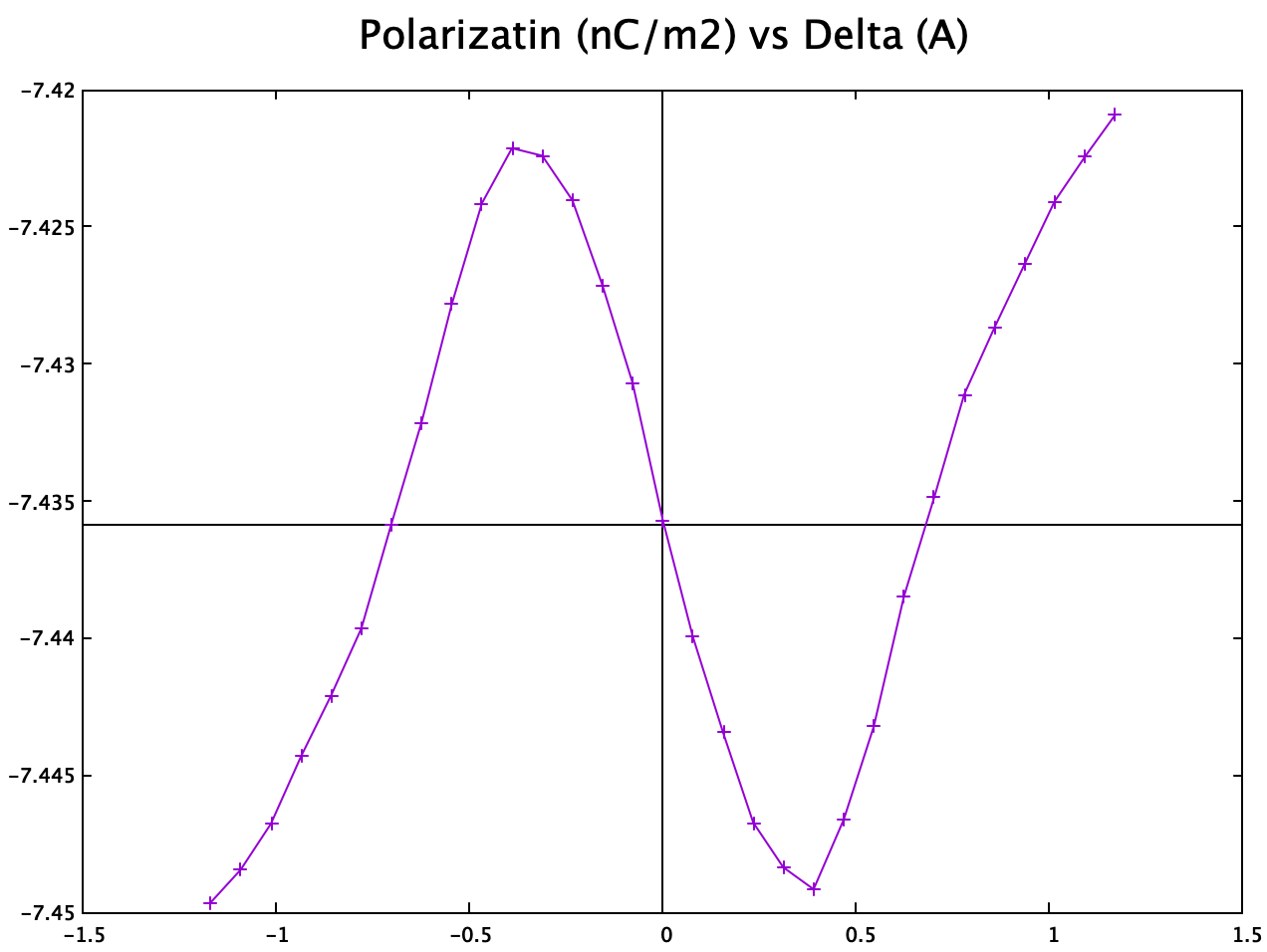Note that the centrosymmetric phase does not have a polarization value of ZERO. Despite the overall trend of the graph is correct, this still puzzles me.

QE 3D system

QE’s example04 strangely did not calculate the polarization of the centrosymmetric phase.

Here, using prototypical BaTiO3 as an example, the total polarization is calculated.

centrosymmetric phase

VALUES OF POLARIZATION
~~~~~~~~~~~~~~~~~~~~~~

The calculation of phases done along the direction of vector 3
of the reciprocal lattice gives the following contribution to
the polarization vector (in different units, and being Omega
the volume of the unit cell):

P =   0.3069997  (mod  15.2444207)  (e/Omega).bohr

P =   0.0007069  (mod   0.0351000)  e/bohr^2

P =   0.0404125  (mod   2.0067288)  C/m^2

The polarization direction is:  ( 0.00000 , 0.00000 , 1.00000 )

Ferroelectric phase

VALUES OF POLARIZATION
~~~~~~~~~~~~~~~~~~~~~~

The calculation of phases done along the direction of vector 3
of the reciprocal lattice gives the following contribution to
the polarization vector (in different units, and being Omega
the volume of the unit cell):

P =   2.4138432  (mod  15.2444207)  (e/Omega).bohr

P =   0.0055578  (mod   0.0351000)  e/bohr^2

P =   0.3177509  (mod   2.0067288)  C/m^2

The polarization direction is:  ( 0.00000 , 0.00000 , 1.00000 )

Total polairzation = 0.3177509-0.0404125 = 0.277 C/m2 (Note that I forgot to add dipole correction to the QE calculations, but it did not hurt the final result much.)

Almost identical to the VASP and experimental value.

Result: QE can be used to calculate total polarization on both periodical and non-periodical directions.

2019-09-09 update

I’ve figured out a way to mimic QE’s occupations = fixed in VASP.

First, do an scf calculation with default number of bands to obtain correct charge density.

Then, using the previous charge density, do an nscf calculatin to obtain berryphase polarization. Note that, we need to excluded any empty bands by fixing the number of bands (NBAND) and occupations.

OR

If the conduction band have minimum overlap with the valence band, simply just change the number of bands to assume a insulator. This usually work for most of the 2D cases if the calculation can be successfully converged.

The results of this procedure are not pretty comparing to the QE and charge center method.

Nevertheless, it works.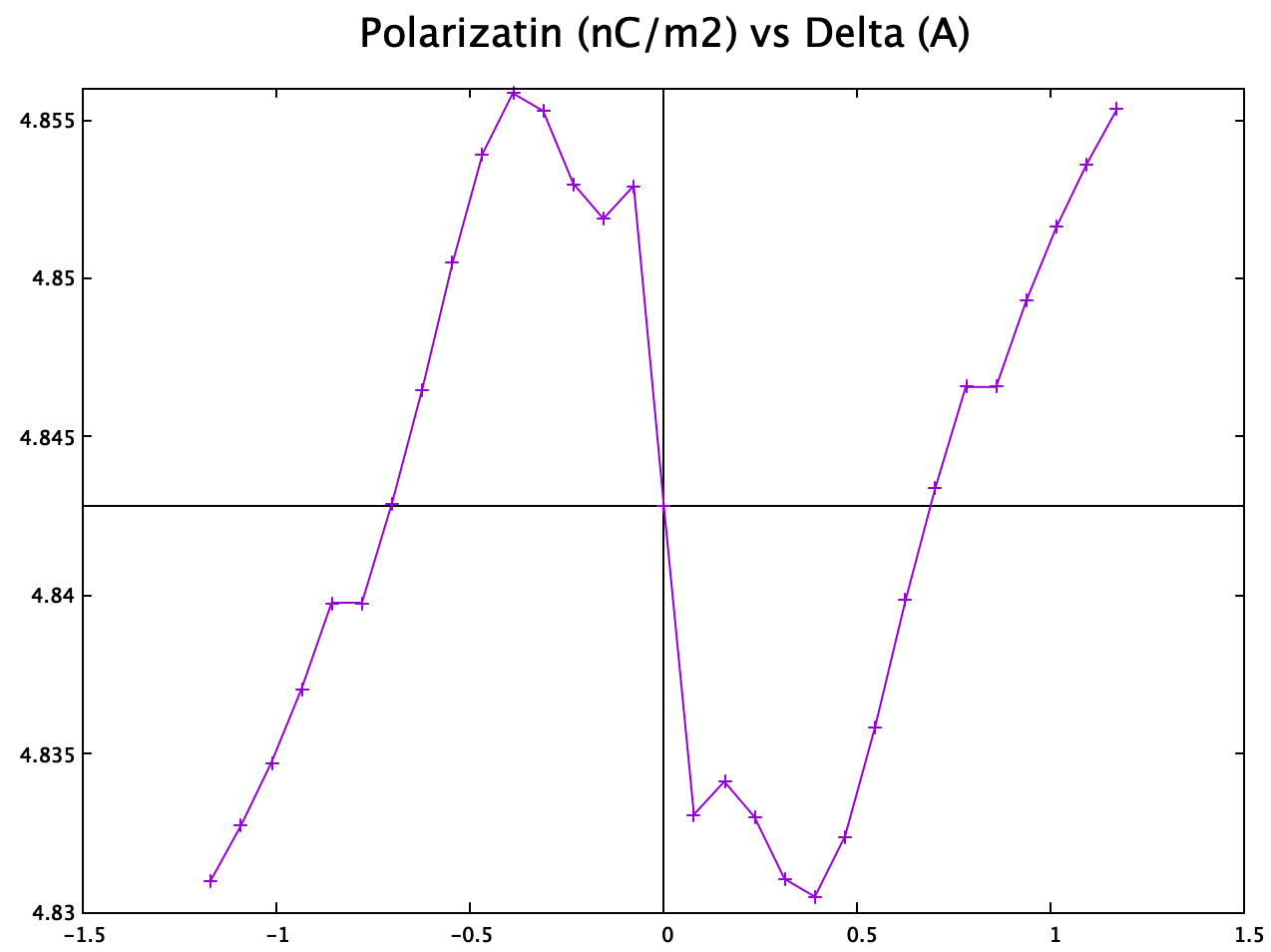UPDATE-2020-03-15 Q: What if we change the centro-symmetric phase for the NbN? Would this behavior (polarization changes sign without structural flip) be a route dependent??

A: No, because the total polarization is smaller than the max opposite polarization, and the Born effective charge (or the slope of the polarization curve) for the flat structure and stable buckled structure are opposite.

But, if the total polarization is larger than the max opposite polarization, qualitatively, this phenomena could change with different centro-symmetric reference structure. However, the polarization curve will still have two turning points.

UPDATE-2020-04-01

1. The position of the dipole layer in VASP is set by (DIPOL+0.5) in fractional coordinates.
2. LVTOT and LVHAR should converge in vacuum. LVTOT converges slower than LVHAR.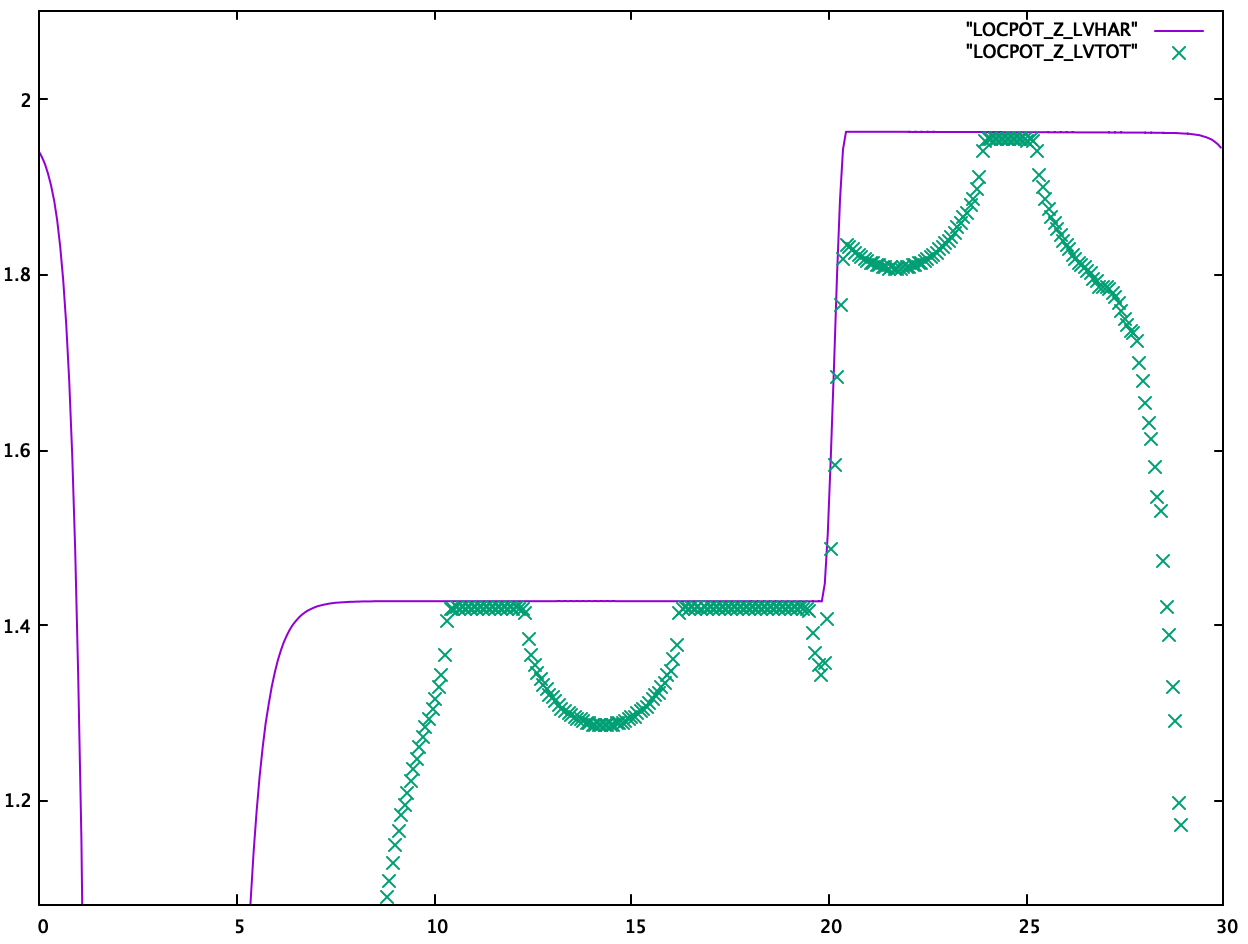Input

I’ve put all input file in a zip file for download: Excel data,VASP, QE.